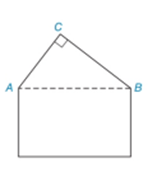Chapter 2.4, Problem 38EElementary Geometry For College St...

7th Edition
Alexander + 2 others
ISBN: 9781337614085

Solutions

Chapter
SectionElementary Geometry For College St...

7th Edition
Alexander + 2 others
ISBN: 9781337614085
Textbook Problem

The roofline of a house shows the shape of a right triangle A B C with m ∠ C = 90 ° . If the measure of ∠ C A B is 24 ° larger than the measure of ∠ C B A , then how large is each angle?To determine

To find:

The value of mCAB and mCBA.

Explanation

Given:

mC=90° and CAB=24°+CBA

Figure (1)

Approach:

The sum of interior angles of a triangle is equal to 180°.

Calculation:

mCAB+mCBA+mC=180°

Substitute 24°+CBA for CAB, and 90° for mC in equation (1).

Still sussing out bartleby?

Check out a sample textbook solution.

See a sample solution

The Solution to Your Study Problems

Bartleby provides explanations to thousands of textbook problems written by our experts, many with advanced degrees!

Get Started

Find more solutions based on key concepts